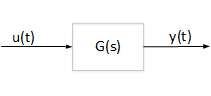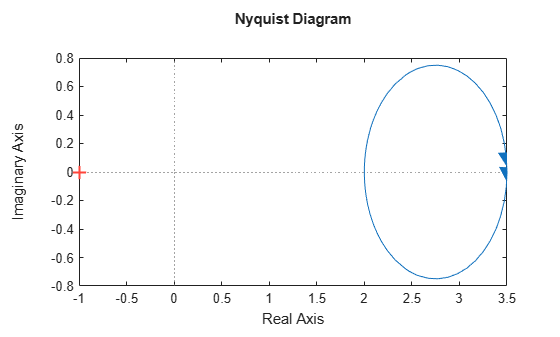# Passivity Indices

This example shows how to compute various measures of passivity for linear time-invariant systems.

### Passive Systems

A linear system G(s) is passive when all I/O trajectories $\left(u\left(t\right),y\left(t\right)\right)$ satisfy

`${\int }_{0}^{T}{y}^{T}\left(t\right)u\left(t\right)dt>0,\phantom{\rule{1em}{0ex}}\forall T>0$`

where ${y}^{T}\left(t\right)$ denotes the transpose of $y\left(t\right)$.To measure "how passive" a system is, we use passivity indices.

• The input passivity index is defined as the largest $\nu$ such that

`${\int }_{0}^{T}{y}^{T}\left(t\right)u\left(t\right)dt>\nu {\int }_{0}^{T}{u}^{T}\left(t\right)u\left(t\right)\right)dt$`

The system G is "input strictly passive" (ISP) when $\nu >0$. $\nu$ is also called the "input feedforward passivity" (IFP) index and corresponds to the minimum feedforward action needed to make the system passive.

• The output passivity index is defined as the largest $\rho$ such that

`${\int }_{0}^{T}\left({y}^{T}\left(t\right)u\left(t\right)dt>\rho {\int }_{0}^{T}{y}^{T}\left(t\right)y\left(t\right)\right)dt$`

The system G is "output strictly passive" (OSP) when $\rho >0$. $\rho$ is also called the "output feedback passivity" (OFP) index and corresponds to the minimum feedback action needed to make the system passive.

• The I/O passivity index is defined as the largest $\tau$ such that

`${\int }_{0}^{T}{y}^{T}\left(t\right)u\left(t\right)dt>\tau {\int }_{0}^{T}\left({u}^{T}\left(t\right)u\left(t\right)+{y}^{T}\left(t\right)y\left(t\right)\right)dt$`

The system is "very strictly passive" (VSP) if $\tau >0$.

### Circuit Example

Consider the following example. We take the current $I$ as the input and the voltage $V$ as the output. Based on Kirchhoff's current and voltage law, we obtain the transfer function for $G\left(s\right)$,

`$G\left(s\right)=\frac{V\left(s\right)}{I\left(s\right)}=\frac{\left(Ls+R\right)\left(Rs+\frac{1}{C}\right)}{L{s}^{2}+2Rs+\frac{1}{C}}.$`Let $R=2$, $L=1$ and $C=0.1$.

```R = 2; L = 1; C = 0.1; s = tf('s'); G = (L*s+R)*(R*s+1/C)/(L*s^2 + 2*R*s+1/C);```

Use `isPassive` to check whether $G\left(s\right)$ is passive.

`PF = isPassive(G)`
```PF = logical 1 ```

Since PF = true, $G\left(s\right)$ is passive. Use `getPassiveIndex` to compute the passivity indices of $G\left(s\right)$.

```% Input passivity index nu = getPassiveIndex(G,'in')```
```nu = 2 ```
```% Output passivity index rho = getPassiveIndex(G,'out')```
```rho = 0.2857 ```
```% I/O passivity index tau = getPassiveIndex(G,'io')```
```tau = 0.2642 ```

Since $\tau >0$, the system $G\left(s\right)$ is very strictly passive.

### Frequency-Domain Characterization

A linear system is passive if and only if it is "positive real":

`$G\left(j\omega \right)+{G}^{H}\left(j\omega \right)>0\phantom{\rule{1em}{0ex}}\forall \omega \in R.$`

The smallest eigenvalue of the left-hand-side is related to the input passivity index $\nu$:

`$\nu =\frac{1}{2}\underset{\omega }{\mathrm{min}}{\lambda }_{\mathrm{min}}\left(G\left(j\omega \right)+{G}^{H}\left(j\omega \right)\right)$`

where ${\lambda }_{\mathrm{min}}$ denotes the smallest eigenvalue. Similarly, when $G\left(s\right)$ is minimum-phase, the output passivity index is given by:

`$\rho =\frac{1}{2}\underset{\omega }{\mathrm{min}}{\lambda }_{\mathrm{min}}\left({G}^{-1}\left(j\omega \right)+{G}^{-H}\left(j\omega \right)\right).$`

Verify this for the circuit example. Plot the Nyquist plot of the circuit transfer function.

`nyquist(G)`The entire Nyquist plot lies in the right-half plane so $G\left(s\right)$ is positive real. The leftmost point on the Nyquist curve is $\left(x,y\right)=\left(2,0\right)$ so the input passivity index is $\nu =2$, the same value we obtained earlier. Similarly, the leftmost point on the Nyquist curve for ${G}^{-1}\left(s\right)$ gives the output passivity index value $\rho =0.286$.

### Relative Passivity Index

It can be shown that the "positive real" condition

`$G\left(j\omega \right)+{G}^{H}\left(j\omega \right)>0\phantom{\rule{1em}{0ex}}\forall \omega \in R$`

is equivalent to the small gain condition

`$||\left(I-G\left(j\omega \right)\right)\left(I+G\left(j\omega \right){\right)}^{-1}||<1\phantom{\rule{1em}{0ex}}\forall \omega \in R.$`

The relative passivity index (R-index) is the peak gain over frequency of $\left(I-G\right)\left(I+G{\right)}^{-1}$ when $I+G$ is minimum phase, and $+\infty$ otherwise:

`$R={‖\left(I-G\right)\left(I+G{\right)}^{-1}‖}_{\infty }.$`

In the time domain, the R-index is the smallest $r>0$ such that

`${\int }_{0}^{T}||y-u|{|}^{2}dt<{r}^{2}{\int }_{0}^{T}||y+u|{|}^{2}dt$`

The system $G\left(s\right)$ is passive if and only if $R<1$, and the smaller $R$ is, the more passive the system is. Use `getPassiveIndex` to compute the R-index for the circuit example.

`R = getPassiveIndex(G)`
```R = 0.5556 ```

The resulting $R$ value indicates that the circuit is a very passive system.